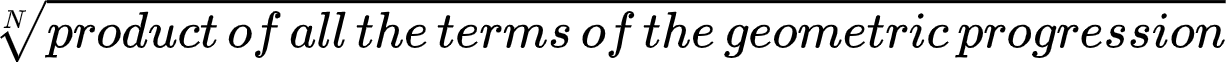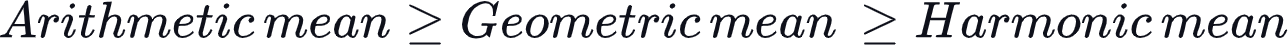New update is available. Click here to update.
Last Updated: Jun 30, 2023

# Progressions - Arithmetic, Geometric and Harmonic progression(AP, GP, HP)0 upvotes

## Introduction

This blog will learn about the progression or sequence and series, which is an important topic for aptitude exams for different roles in detail and then build a better understanding of these concepts with the help of some sample problems

So let's first begin with a brief definition of progression when numbers are arranged according to some definite rule to predict the next sequence term. Such arrangements are called progressions.

There are three major types of progressions we need to learn-

• Arithmetic progression.
• Geometric progressions.
• Harmonic progressions.

So let’s learn about them one by one-

## Arithmetic progression(AP)

Arithmetic progression is a sequence of numbers in which the difference between any two consecutive terms is constant. The difference between the consecutive terms for the sequence is called a common difference, and we can add it to any term of the sequence to find the next term.

For example- 1, 3, 5, 7, 9, 11 is an arithmetic progression as the difference between any two consecutive terms is constant, i.e., 3-1=5-3=7-5=9-7=11-9=2.

### Important formulas for arithmetic progression

Let, A be the first term, D be a common difference, and N be the number of terms in the sequence.

1. The nth term of the arithmetic progression= A+(N-1)D
2. Sum of N terms of the arithmetic progression= 1⁄2(N)(A+last term)=1⁄2(N)(2*A +(N-1)*D)
3. Arithmetic mean = sum of all the terms in the arithmetic progression/ N

## Geometric progression(GP)

Geometric progression is a sequence of numbers in which the ratio between any two consecutive terms is constant. The constant ratio between the consecutive terms for the sequence is called the common ratio, and we can multiply it to any term of the sequence to find the next term.

For example- 2, 4, 8, 16, 32, 64  is a geometric progression as the ratio between any two consecutive terms is constant, i.e., 64/32=32/16=16/8=8/4=4/2=2.

### Important formulas for geometric progressions

Let, A be the first term, R be the common ratio, and N be the number of terms in the sequence.

1. The nth term of the geometric progressions= A*RN-1
2. Sum of N terms of the geometric progressions-
1. If common ratio<1, Sum= A(1-(R)N)/(1-R)
2. If common ratio<1, Sum= A((R)N-1)/(R-1)
3. For infinite geometric progression(common ratio<1), Sum=A/(1- R)
3. Geometric mean =## Harmonic progression(HP)

Harmonic progression is a sequence of numbers in which the multiplicative inverse or reciprocal of the terms forms an arithmetic progression.

For example- 1, 3, 5, 7, 9, 11 is an arithmetic progression. Thus 1, 1⁄3, 1⁄5, 1⁄7, 1⁄9, 1⁄11 will be a harmonic progression. Inharmonic progression, the difference between reciprocals of any two consecutive terms is constant.

### Important formulas for harmonic progressions

Let, A be the reciprocal of the first term of the harmonic progression, D be the common difference between the reciprocals of any two consecutive terms, and N be the number of terms in the sequence.

1. Nth term of the harmonic progressions= 1/(A+(N-1)D)
2. Sum of N terms of the harmonic progressions= 2/N(2A+(N-1)D)
3. Harmonic mean =N/(1/A+1/(A+D)......+1/(last term))

## Sample problems

Let’s see some problems to build a better understanding of these concepts-

Problem-1 Find the common difference of the following AP and write the AP up to 8 terms.

7, 12, 17, 22, 27...

Solution- The common difference for an AP is the difference between any of its two consecutive terms. Thus for this AP, the common difference will be 12-7=5.

Since we are given the five terms of the AP, we can find the next terms by adding the common difference.

6th term= 27+5=32

7th term= 32+5=37

8th term= 37+5=42

Thus the AP is 7, 12, 17, 22, 27, 32, 37, 42

Problem-2 If a, b, c are in AP, find the value of a-2b+c.

Solution- Since a, b, c are in AP. Let's suppose the common difference of this AP is d.

Then the b=a+d, c=a+2d.

Now putting the value of b and c, in the expression a-2b+c, it will become a-2(a+d)+a+2d

=a-2a-2d+a+2d=0

Thus, the value of the expression a-2b+c will be zero if a, b, c are in AP.

Problem-3 The sum of the first six terms of an AP is 96, and the common difference is 4. Find the 4th term of the AP.

Solution- Let, the given AP has the first term as A, and it is given that total no. of terms=6, and the common difference is 4. Now, putting these values in the formula for the sum of an AP-

1⁄2(N)(2*A +(N-1)*D)= 6/2(2*A+5*4).

Since it is given that the sum of the AP is 96

=> 6/2(2*A+5*4)=96

=> A=6.

Thus, the required AP is 6, 10, 14, 18, 22. 26. The 4th term of the AP is 18.

Problem-4 Find the number of terms in the following GP and also find its sum.

2, 6, 18, …., 1458.

Solution- To find the number of terms in the GP, we first need to find the common ratio of the progression. Since common ratio(R) = Tn+1/Tn=6/2=3.

Now, we also know that Nth term of a GP is A*RN-1 where R is the common ratio.

Since the last term of the given GP is 1458, Now, equate it with the above formula to find the number of terms in the progression-

=>  1458= A*RN-1

=>  2*729= 2*3N-1

=>  2*36= 2*3N-1

On comparing LHS with RHS

=>  6=N-1

=>  N=7

Problem-5 If the sum of reciprocals of the first 13 terms of an HP is 260, find the 7th term of the sequence.

Solution- Sum of reciprocals of an HP refers to the sum of AP used to form the HP,

Let’ the first term of the AP be A, and common difference be D, then the sum of the AP will be 1⁄2(13)(2*A +(13-1)*D) Since the sum is given to be 260.

=>  1⁄2(13)(2*A +(13-1)*D)=260

=>  2*A +(13-1)*D=40

=>  2*A +12*D=40

=>  A +6*D=20

Since the Nth term of an HP is 1/(A+(N-1)D)

Thus, the 7th term of given HP will be 1/(A+6*D)=1/20.

Q1. How can we check if the given sequence is an AP?

We can check if a sequence is an AP or not by comparing the consecutive terms of the sequence. If the difference between any two consecutive terms is constant, then the sequence is an arithmetic progression.

Q2. How to check if the given sequence is a GP?

We can check if a sequence is a GP or not by comparing the consecutive terms of the sequence. If the ratio between any two consecutive terms is constant, then the sequence is a geometric progression.

Q3. How to check if the given sequence is an HP?

We can check if a sequence is an HP or not by finding the reciprocals of its terms; if these reciprocals form an AP, the sequence is a harmonic progression.

Q4. What is the relation between Arithmetic mean, Geometric mean, and Harmonic mean?

The relation between Arithmetic mean Geometric mean, and Harmonic mean is as follows-Q5. Find the scenario in which all three values, i.e., Arithmetic mean, Geometric mean, and Harmonic mean for a sequence, can be equal?

Arithmetic, geometric, and Harmonic mean for a sequence can be equal when all the terms in the sequence are equal.

## Key takeaways

This blog has discussed the important formulas and concepts regarding the progression, as it is one of the most important topics for aptitude exams.-

We started with discussing the arithmetic progression, we learned the formulas to find the Nth term, the sum of the sequence up to the nth term, and the arithmetic mean of a sequence. Then we learned similar formulas for geometric and harmonic progressions as well. Lastly, we tried some problems to develop a better understanding of these concepts.